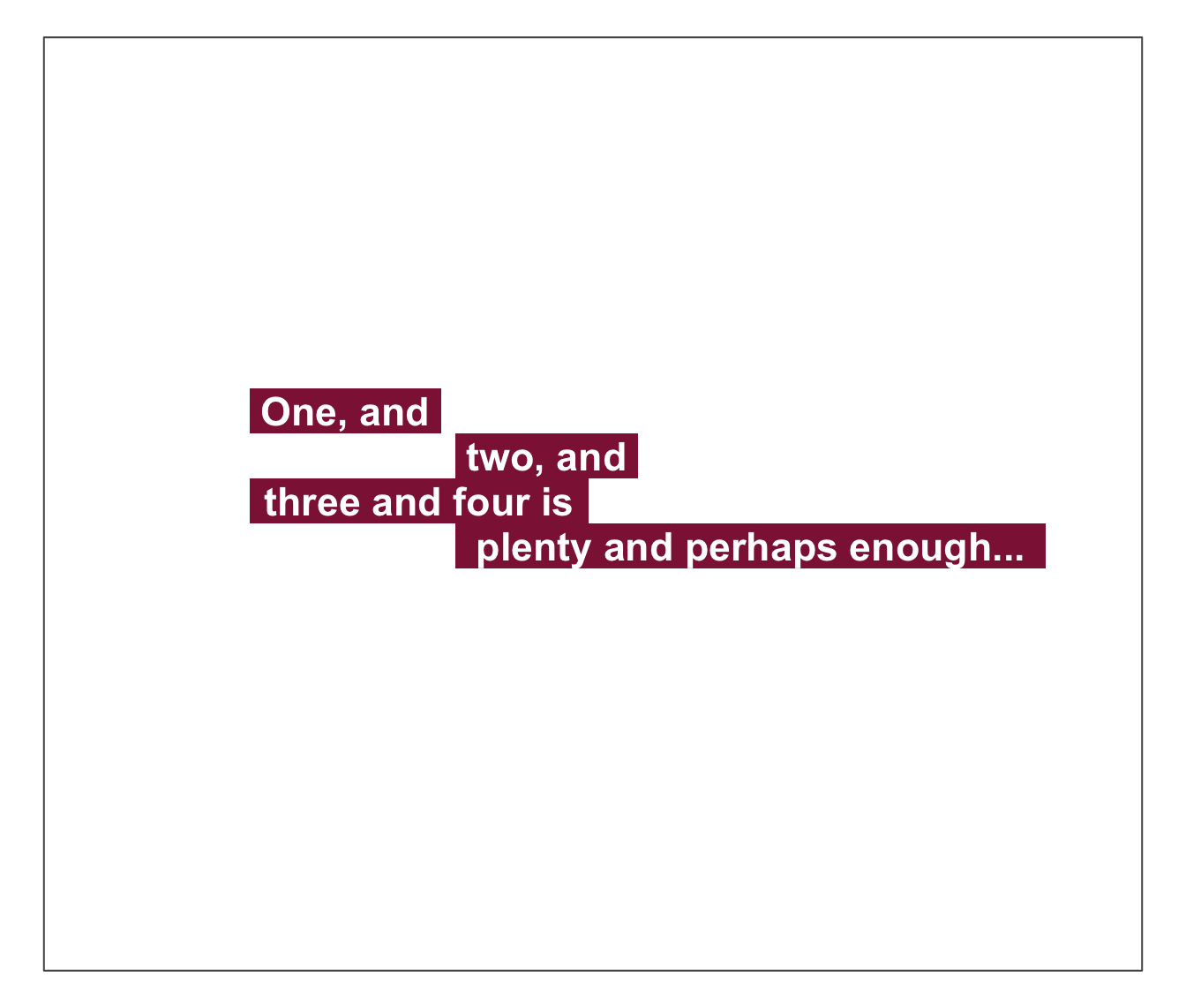mark plots 1 or more text strings (provided as a character vector labels) to an (existing or new) plot and places a colored box behind each label to mark it (i.e., highlight or make it stand out from the background).

mark(
labels,
x = 0,
y = 0.55,
x_layout = NA,
y_layout = "even",
col = "black",
col_bg = Seeblau,
cex = 2,
font = 2,
new_plot = "none"
)

## Arguments

labels A character vector specifying the text labels to be plotted. A numeric vector of x-coordinates at which the text labels in labels should be written. If the lengths of x and y differ, the shorter one is recycled. Default: x = 0. A numeric vector of y-coordinates at which the text labels in labels should be written. If the lengths of x and y differ, the shorter one is recycled. Default: y = .55. An optional numeric vector or character string to control the horizontal positions of labels. Numeric values are interpreted as increments to values of x and recycled (to enable stepwise or alternating patterns). 3 character string options are: "center" (i.e., center wrt. first label or plot center), "left" (i.e., left wrt. first label or plot center), "right" (i.e., right wrt. first label or plot center). Default: x_layout = NA (i.e., using values of x). A numeric value or character string to control the vertical positions of labels. Numeric values are interpreted as increments to values of y and recycled (to enable stepwise or alternating patterns). 2 character string options are: "even" (i.e., even distribution of labels across available y-space) and "flush" (i.e., no space between adjacent labels, i.e., y_layout = 0). Default: y_layout = "even". The color(s) of the text label(s). Default: col_lbl = "black". The color(s) to highlight or fill the rectangle(s) with. Default: col_bg = Seeblau. Numeric character expansion factor(s), multiplied by par("cex") to yield the character size(s). Default: cex = 2. The font type(s) to be used. Default: font = 2 (i.e., bold). Should a new plot be generated? Set to "blank" or "slide" to create a new plot. Default: new_plot = "none" (i.e., add to an existing plot).

## Details

The positions of the text elements in labels can be specified by providing their coordinates (as x and y arguments) or by providing an initial position and an y_layout (see below).

Text formatting parameters (like col, col_bg, cex, font) are recycled to match length(labels).

mark uses the base graphics system graphics::.

slide and xbox to create simple plots (without text).

Other text functions: post(), uline(), url_unikn()

## Examples

# Basics:
mark(labels = "This is a test.", new_plot = "blank")  # create a new blank plot
mark(labels = "More testing here...", y = .45, col_bg = pal_pinky[])  # add to plot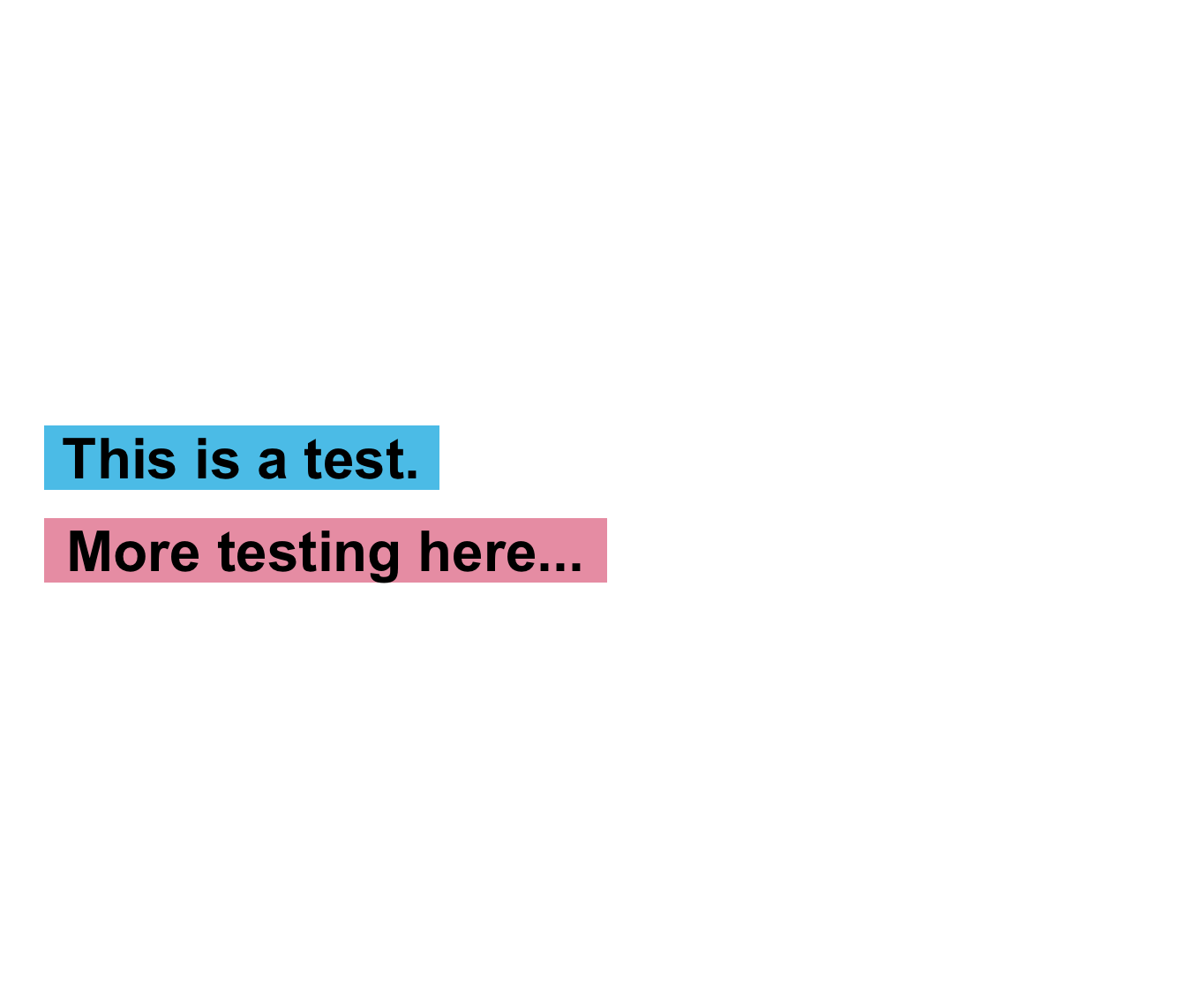# Example:
# (a) Mark text on an existing plot:
plot(x = 0, y = 0, type = "n", xlim = c(0, 1), ylim = c(0, 1), xlab = "", ylab = "")
mark(x = 0, y = .8, labels = "Mark (on an existing plot)")  # uses existing plot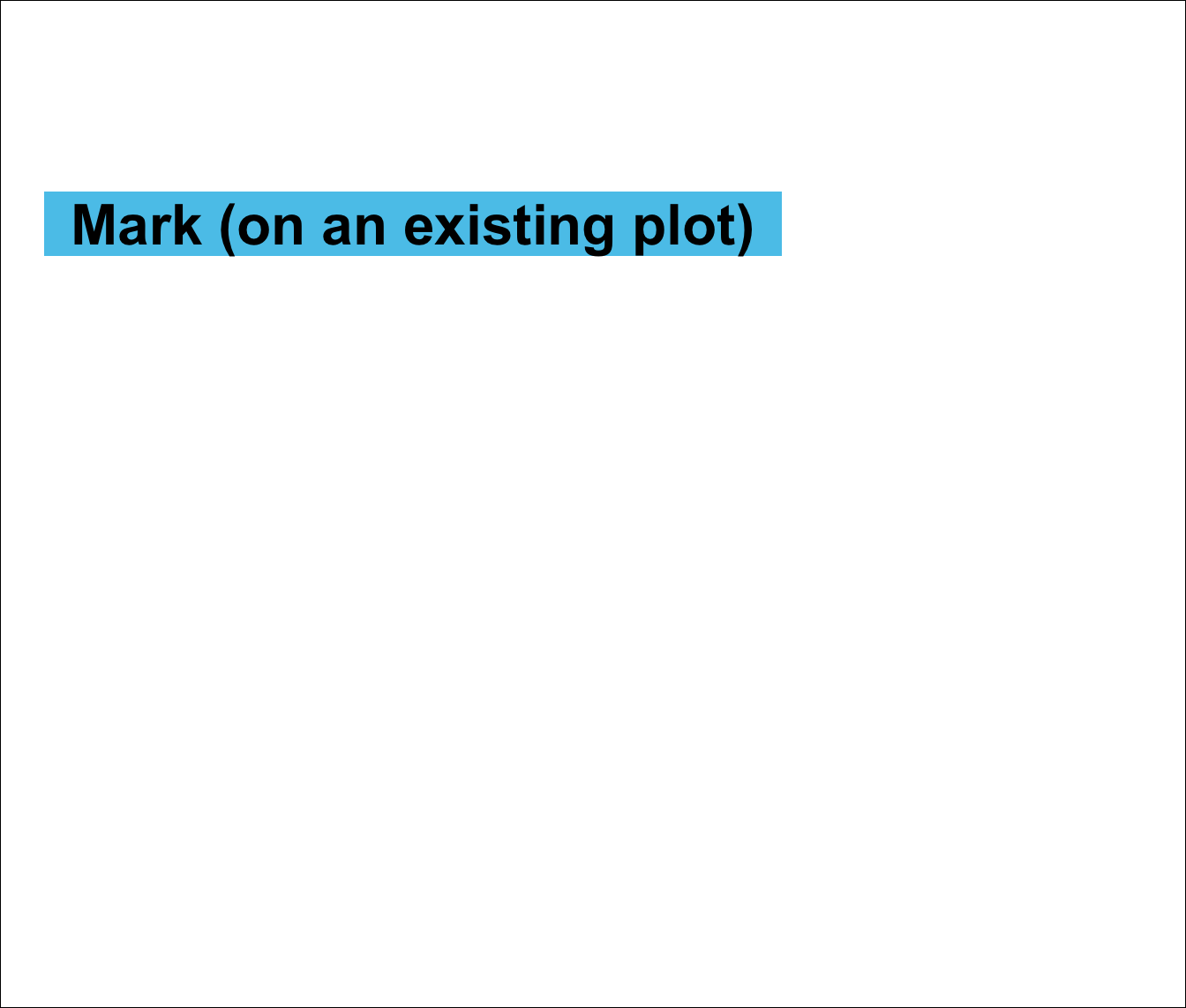# (b) Mark text on a new plot:
mark(x = 0, y = .8, labels = "Mark (and create a new plot)",
new_plot = "slide")  # starts a new plot

# (c) More text and decorations:
mark(x = 0, y = c(.60, .50),
labels = c("Highlighting text is simple", "and effective"),
cex = 1.5, col_bg = c(pal_seeblau[], pal_seeblau[]))

mark(labels = c("It is also flexible", "but to be handled with care"),
x = .4, y = .3, y_layout = "flush", cex = 1.2,
col = c("white", "black"), col_bg = c(pal_seeblau[], "gold"))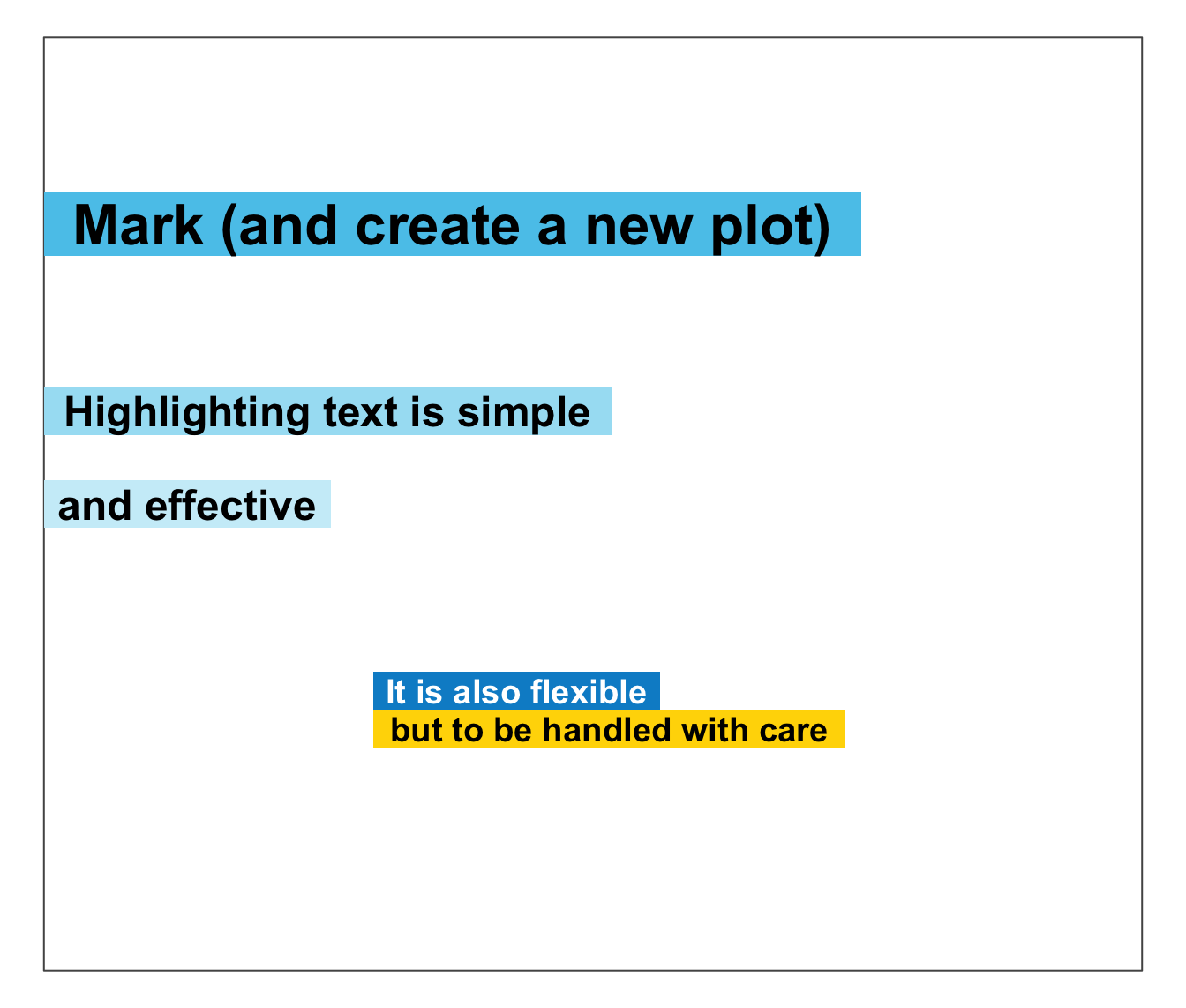# Using x_layout and y_layout:
mark(labels = c("Ene,", "mene, miste,", "es rappelt", "in der Kiste."),
cex = 1.4, font = 2, col = "white", col_bg = Petrol,
x = NA, y = .85, x_layout = "center", y_layout = "even", new_plot = "slide")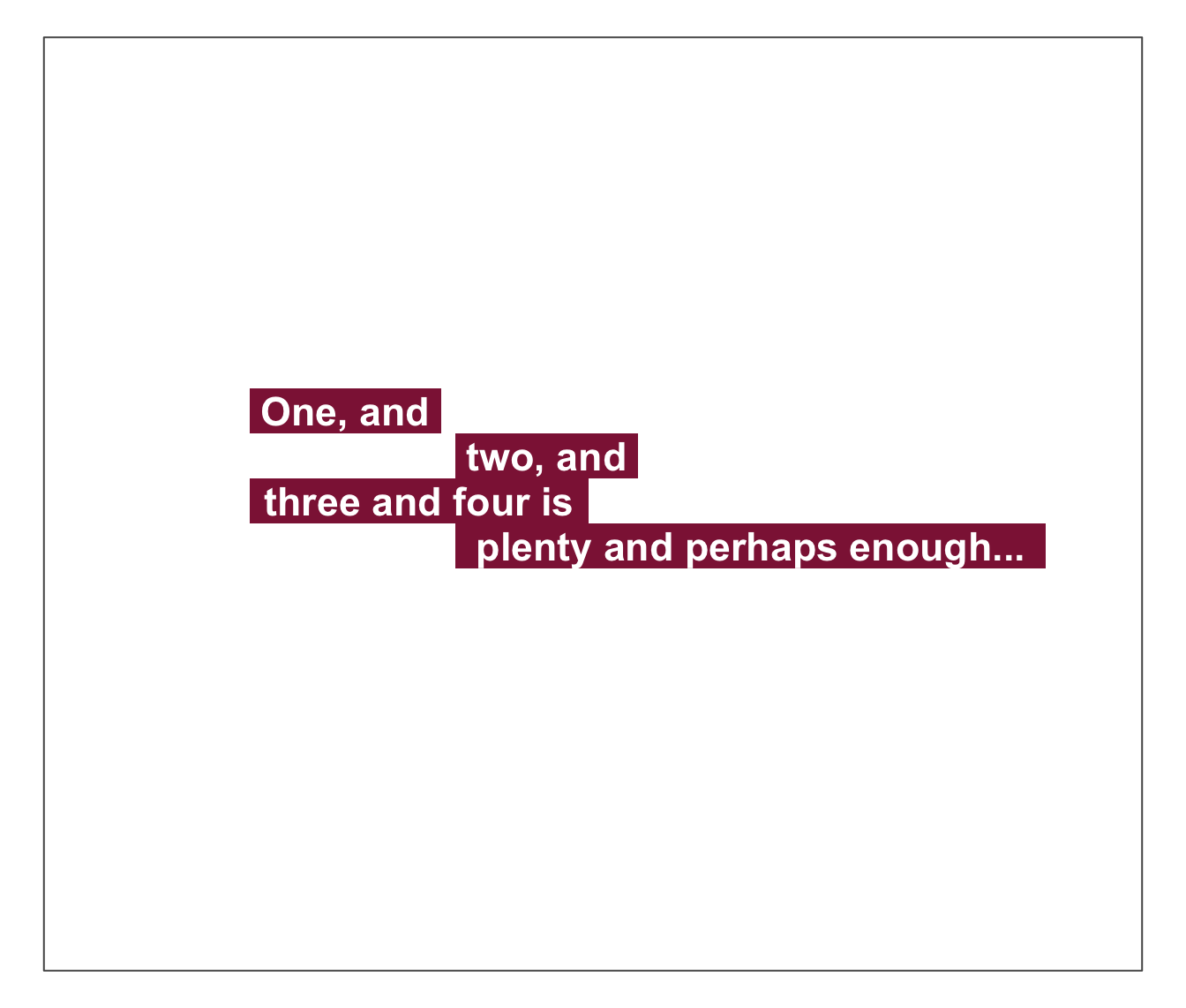mark(labels = c("One, and", "two, and", "three and four is", "plenty and perhaps enough..."),
cex = 1.4, font = 2, col = "white", col_bg = Bordeaux,
x = .5, y = .6, x_layout = c(-.25, +.25), y_layout = 0, new_plot = "slide")
#> Warning: the condition has length > 1 and only the first element will be used